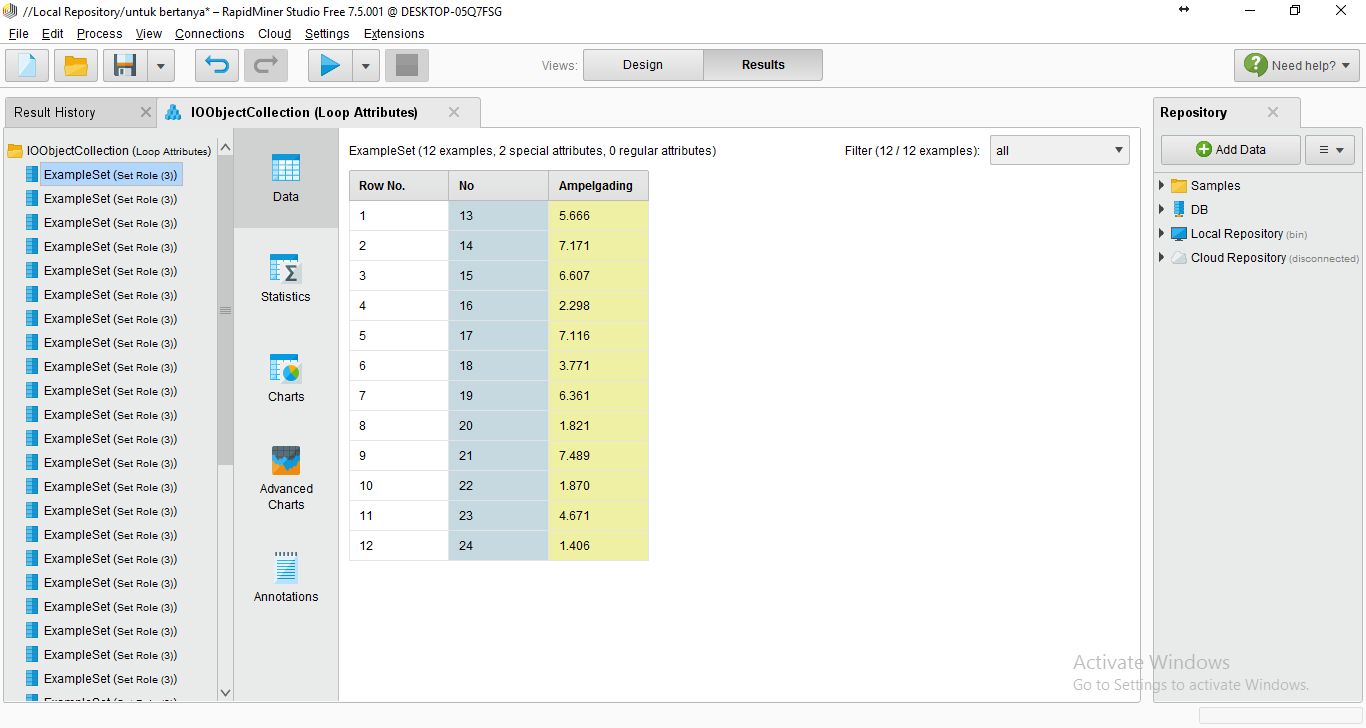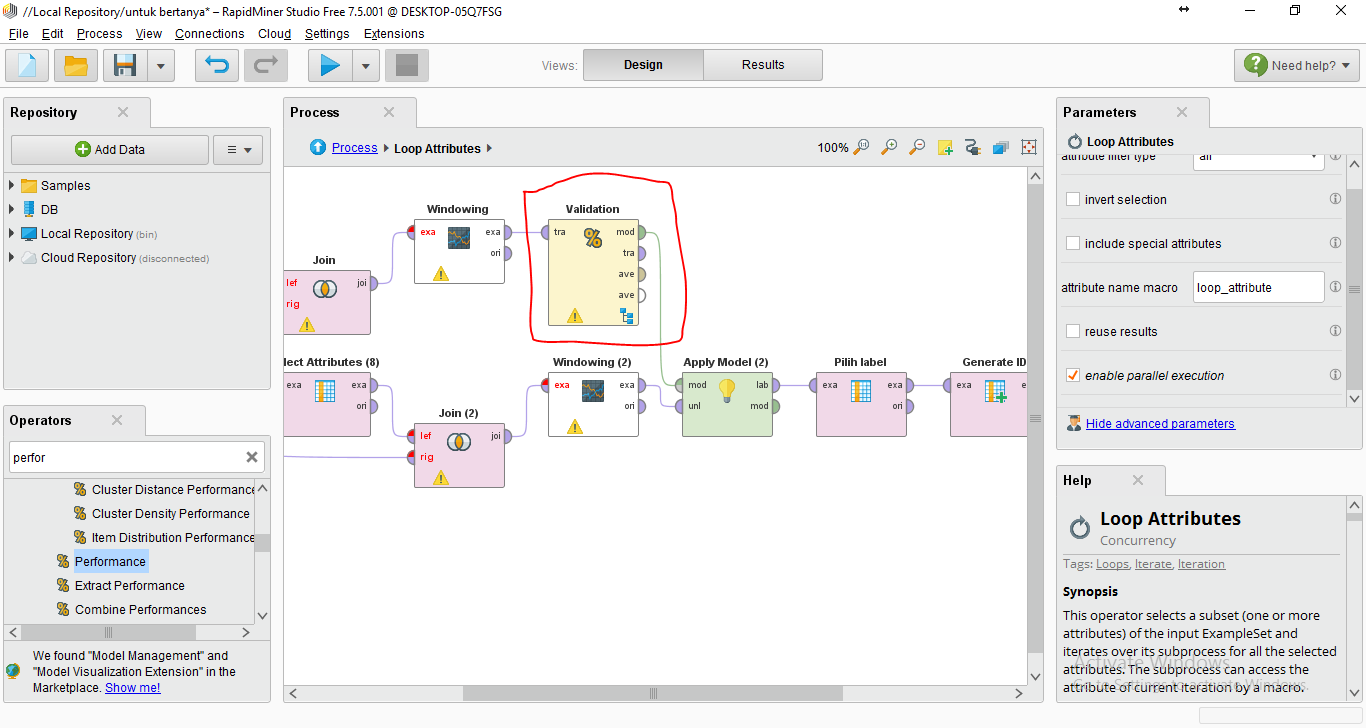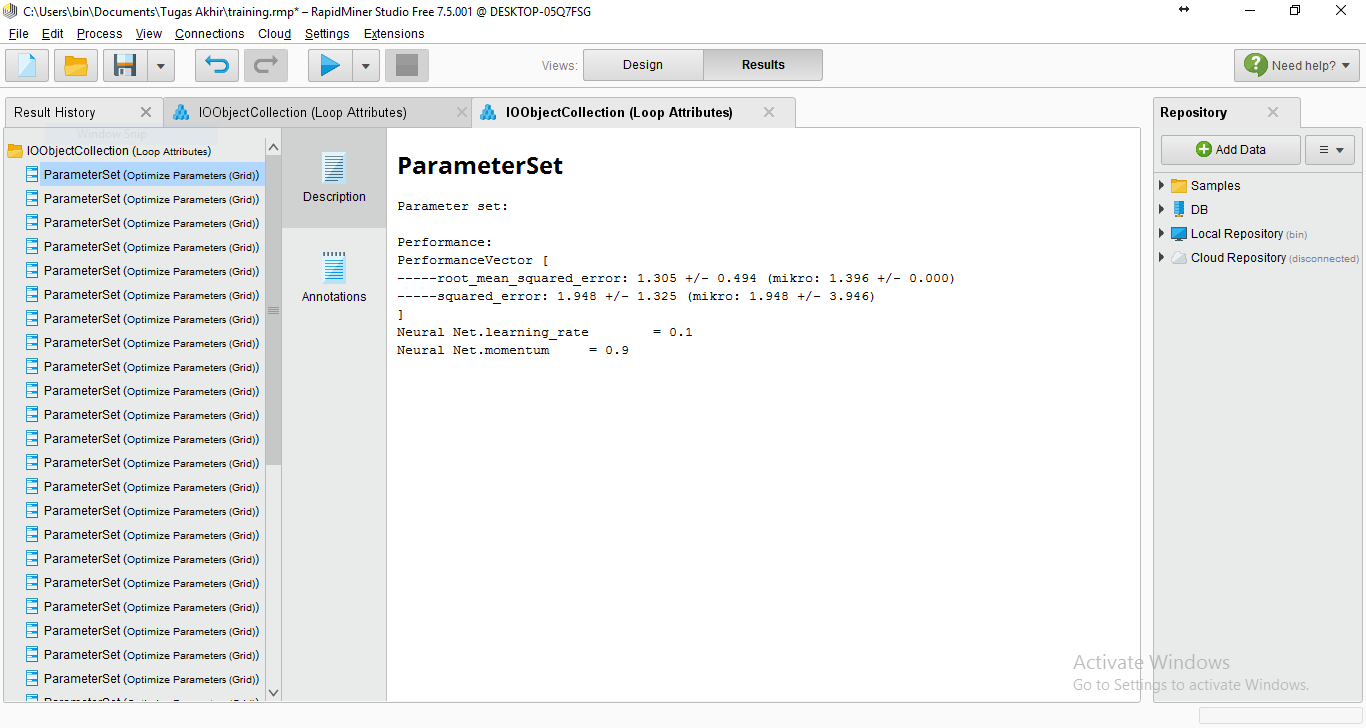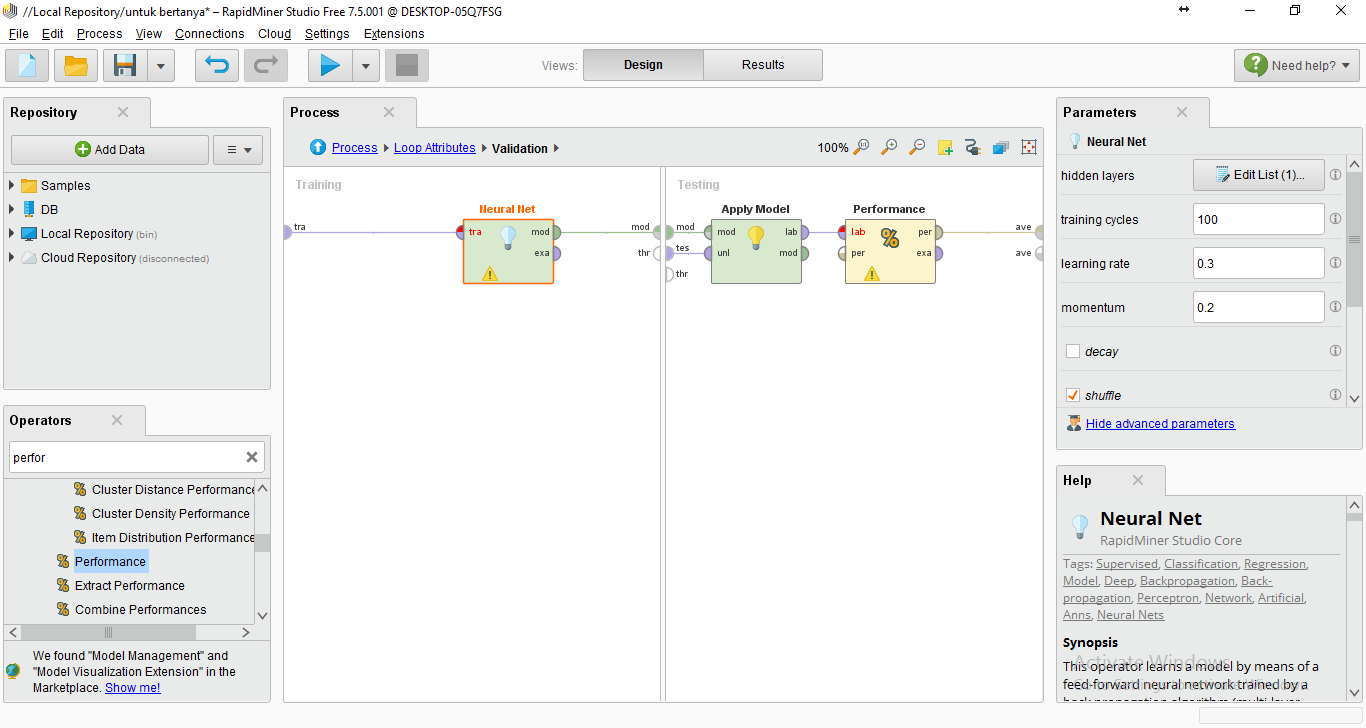# How to apply different neural network model inside loop attribute?

Member Posts: 46Guru
edited November 2018 in Help

hello everyone, im working with neural network for forecasting

in my project, i got IO Object Collection that contain example sets from loop attributes.Example Sets on IO Object Collection

Inside loop attributes, i put validation operators to forecast with neural network operator so each example set have their own prediction.sliding window validation

To get the best model for each example set, i used optimization (grid) operator and each example set got their own best model (different training cycle, learning rate and momentum).Result on Optimization Parameter Grid (on each example)

When i got their own best model, how do i apply the result of optimization parameter to each example sets in my process? because if i put it on neural net operator, it only apply an model.inside validation operator

• Member Posts: 46Guru
Solution Accepted

excuse me sir, when i put write model operator after optimize parameter (grid) operator, is the model that come out from mod port is the best model? if its yes, the model name parameter i set test_%{loop_attribute} and the result is i got .mod file for each loop, so i can apply it with read model and connect it to apply model for testing• RapidMiner Certified Analyst, RapidMiner Certified Expert, Member Posts: 1,761Unicorn
Solution Accepted

That is correct, the model that comes out of the Optimize Parameters operator is the best model.

• RapidMiner Certified Analyst, RapidMiner Certified Expert, Member Posts: 1,761Unicorn

Couldn't you store each model right after the validation and save it with a macro? like model_%{loop_attribute} ? This way you can store each iteration of the model and then use it to score your individual data sets later.

• Member Posts: 46Guru

yeah, i've tried the macro to save the model, i put it adter validation operator, but the name of the model is always overwrite and i dont know what values should i fill on it. so its not possible to appy if they have different amount of nodes in hidden layer?

• RapidMiner Certified Analyst, RapidMiner Certified Expert, Member Posts: 1,761Unicorn

To prevent it from overwriting, you need to use a valid macro. I choose %{loop_attribute} because it's already defined by the Loop Attribiutes operator. Another option is to use %{t} which is the timestamp when it gets executed.

• Member Posts: 46Guru

im sorry i still confusing with your explanation

• RapidMiner Certified Analyst, RapidMiner Certified Expert, Member Posts: 1,761Unicorn

You can save the model after each iteration provided that you append the model name with a macro like the timestamp. You would need to save it as "mymodel_%{t}" this way it doesn't overwrite it.

• Member Posts: 46Guru

instead of using macro, is it possible if i made a list of value parameter of ANN model for each example sets and apply it in my process and made each example sets recognize their model? i've tried write parameter operator but the problem is the values overwrite with the latest loop_attribute and if the problem resolved how to apply the result of write parameter operator on my process?

• RapidMiner Certified Analyst, RapidMiner Certified Expert, Member Posts: 1,761Unicorn

Have you thought of using the Recall and Remember operator? You might be able to load in the write parameters in a loop, Remember them for the one iteration and then load in the second one for hte second iteration.

• Member Posts: 46Guru

Sorry, I'm more confused
I'm going to edit my topic and add pictures to make it easy to understand and know where to add operator

• Member Posts: 46Guru

and i didn't find any solution yetim so confused

• RapidMiner Certified Analyst, RapidMiner Certified Expert, Member Posts: 1,761Unicorn

An optimized model with the LR and MOM values are exported with each parameter optimization. I see that you have a lot of them in your output because of the loop and using them is going to be tricky but I don't think that hard.

I would consider scoring your data right after the parameter optimization. In this way you would loop your training and optimization and then simulatenously score your data inside the loop, then save each score set with a macro or use annotation to differeniate them.

• Member Posts: 46Guru

yeah i got lot of them because its the best parameter for each example set that i got from loop and join. but i need to know the trick to apply the model that i got because it is the core of the project.

Should i really use macro operator? because when i got best model from optimization parameter operator, i will remove optimization parameter operator and swtich with validation operator which was previously inside optimization parameter operator and apply the model that i got and apply the mod port to data testing.

• Member Posts: 46Guru

up

• Member Posts: 46Guru

so... its not possible?

• RapidMiner Certified Analyst, RapidMiner Certified Expert, Member Posts: 1,761Unicorn

It's probably possible but very complex. I would post your process and data here and see if someone wants to take up the challenge. Actually, we're starting data challenges if you want to throw out a prize like here: http://community.rapidminer.com/t5/General-Chit-Chat/RapidMiner-Data-Modeling-CHALLENGE-200-in-cash-and-prizes/m-p/39159

• RapidMiner Certified Analyst, RapidMiner Certified Expert, Member Posts: 1,761Unicorn

I just realized you did post your process and data.

Something like this doesn't work?

`<?xml version="1.0" encoding="UTF-8"?><process version="7.5.001">  <context>    <input/>    <output/>    <macros/>  </context>  <operator activated="true" class="process" compatibility="7.5.001" expanded="true" name="Process">    <parameter key="encoding" value="SYSTEM"/>    <process expanded="true">      <operator activated="true" class="read_csv" compatibility="7.5.001" expanded="true" height="68" name="Read CSV" width="90" x="45" y="34">        <parameter key="csv_file" value="C:\Users\ThomasOtt\Downloads\Training (2).csv"/>        <parameter key="column_separators" value=","/>        <parameter key="first_row_as_names" value="false"/>        <list key="annotations">          <parameter key="0" value="Name"/>        </list>        <list key="data_set_meta_data_information">          <parameter key="0" value="No.true.integer.attribute"/>          <parameter key="1" value="Bulan.true.polynominal.attribute"/>          <parameter key="2" value="Tahun.true.integer.attribute"/>          <parameter key="3" value="Ampelgading.true.integer.attribute"/>          <parameter key="4" value="Ardimulyo.true.integer.attribute"/>          <parameter key="5" value="Bantur.true.integer.attribute"/>          <parameter key="6" value="Bululawang.true.integer.attribute"/>          <parameter key="7" value="Dampit.true.integer.attribute"/>          <parameter key="8" value="Dau.true.integer.attribute"/>          <parameter key="9" value="Donomulyo.true.integer.attribute"/>          <parameter key="10" value="Gedangan.true.integer.attribute"/>          <parameter key="11" value="Gondanglegi.true.integer.attribute"/>          <parameter key="12" value="Jabung.true.integer.attribute"/>          <parameter key="13" value="Kalipare.true.integer.attribute"/>          <parameter key="14" value="Karangploso.true.integer.attribute"/>          <parameter key="15" value="Kasembon.true.integer.attribute"/>          <parameter key="16" value="Kepanjen.true.integer.attribute"/>          <parameter key="17" value="Ketawang.true.integer.attribute"/>          <parameter key="18" value="Kromengan.true.integer.attribute"/>          <parameter key="19" value="Lawang.true.integer.attribute"/>          <parameter key="20" value="Ngajum.true.integer.attribute"/>          <parameter key="21" value="Ngantang.true.integer.attribute"/>          <parameter key="22" value="Pagak.true.integer.attribute"/>          <parameter key="23" value="Pagelaran.true.integer.attribute"/>          <parameter key="24" value="Pakis.true.integer.attribute"/>          <parameter key="25" value="Pakisaji.true.integer.attribute"/>          <parameter key="26" value="Pamotan.true.integer.attribute"/>          <parameter key="27" value="Poncokusumo.true.integer.attribute"/>          <parameter key="28" value="Pujon.true.integer.attribute"/>          <parameter key="29" value="Singosari.true.integer.attribute"/>          <parameter key="30" value="Sitiarjo.true.integer.attribute"/>          <parameter key="31" value="Sumbermanjing Kulon.true.integer.attribute"/>          <parameter key="32" value="Sumbermanjing Wetan.true.integer.attribute"/>          <parameter key="33" value="Sumberpucung.true.integer.attribute"/>          <parameter key="34" value="Tajinan.true.integer.attribute"/>          <parameter key="35" value="Tirtoyudo.true.integer.attribute"/>          <parameter key="36" value="Tumpang.true.integer.attribute"/>          <parameter key="37" value="Turen.true.integer.attribute"/>          <parameter key="38" value="Wagir.true.integer.attribute"/>          <parameter key="39" value="Wajak.true.integer.attribute"/>          <parameter key="40" value="Wonokerto.true.integer.attribute"/>          <parameter key="41" value="Wonosari.true.integer.attribute"/>          <parameter key="42" value="Suhu.true.real.attribute"/>          <parameter key="43" value="Curah Hujan.true.integer.attribute"/>          <parameter key="44" value="Kelembaban.true.real.attribute"/>        </list>      </operator>      <operator activated="true" class="set_role" compatibility="7.5.001" expanded="true" height="82" name="Set Role" width="90" x="179" y="34">        <parameter key="attribute_name" value="No"/>        <parameter key="target_role" value="id"/>        <list key="set_additional_roles"/>      </operator>      <operator activated="true" class="select_attributes" compatibility="7.5.001" expanded="true" height="82" name="Select Attributes (4)" width="90" x="313" y="34">        <parameter key="attribute_filter_type" value="regular_expression"/>        <parameter key="attributes" value="No|Ampelgading|Ardimulyo|Bantur|Bululawang|Curah Hujan|Dampit|Dau|Donomulyo|Gedangan|Gondanglegi|Jabung|Kalipare|Karangploso|Kasembon|Kelembaban|Kepanjen|Ketawang|Kromengan|Lawang|Ngajum|Ngantang|Pagak|Pagelaran|Pakis|Pakisaji|Pamotan|Poncokusumo|Pujon|Singosari|Sitiarjo|Suhu|Sumbermanjing Kulon|Sumbermanjing Wetan|Sumberpucung|Tajinan|Tirtoyudo|Tumpang|Turen|Wagir|Wajak|Wonokerto|Wonosari"/>        <parameter key="regular_expression" value="Bulan|Tahun"/>        <parameter key="invert_selection" value="true"/>      </operator>      <operator activated="true" class="multiply" compatibility="7.5.001" expanded="true" height="103" name="Multiply" width="90" x="447" y="34"/>      <operator activated="true" class="select_attributes" compatibility="7.5.001" expanded="true" height="82" name="Select Attributes (3)" width="90" x="648" y="136">        <parameter key="attribute_filter_type" value="regular_expression"/>        <parameter key="attributes" value="Wonosari|Wonokerto|Wajak|Wagir|Turen|Tumpang|Tirtoyudo|Tajinan|Sumberpucung|Sumbermanjing Wetan|Sumbermanjing Kulon|Sitiarjo|Singosari|Pujon|Poncokusumo|Pamotan|Pakisaji|Pakis|Pagelaran|Pagak|Ngantang|Ngajum|Lawang|Kromengan|Ketawang|Kepanjen|Kasembon|Karangploso|Kalipare|Jabung|Gondanglegi|Gedangan|Donomulyo|Dau|Dampit|Bululawang|Bantur|Ardimulyo|Ampelgading"/>        <parameter key="regular_expression" value="No|Suhu|Curah Hujan|Kelembaban"/>      </operator>      <operator activated="true" class="select_attributes" compatibility="7.5.001" expanded="true" height="82" name="Select Attributes" width="90" x="648" y="34">        <parameter key="attribute_filter_type" value="regular_expression"/>        <parameter key="attributes" value="Wonosari|Wonokerto|Wajak|Wagir|Turen|Tumpang|Tirtoyudo|Tajinan|Sumberpucung|Sumbermanjing Wetan|Sumbermanjing Kulon|Sitiarjo|Singosari|Pujon|Poncokusumo|Pamotan|Pakisaji|Pakis|Pagelaran|Pagak|Ngantang|Ngajum|Lawang|Kromengan|Ketawang|Kepanjen|Kasembon|Karangploso|Kalipare|Jabung|Gondanglegi|Gedangan|Donomulyo|Dau|Dampit|Bululawang|Bantur|Ardimulyo|Ampelgading"/>        <parameter key="regular_expression" value="No|Suhu|Curah Hujan|Kelembaban"/>        <parameter key="invert_selection" value="true"/>      </operator>      <operator activated="true" class="concurrency:loop_attributes" compatibility="7.5.001" expanded="true" height="124" name="Loop Attributes" width="90" x="849" y="34">        <process expanded="true">          <operator activated="true" class="select_attributes" compatibility="7.5.001" expanded="true" height="82" name="Select Attributes (5)" width="90" x="179" y="34">            <parameter key="attribute_filter_type" value="single"/>            <parameter key="attribute" value="%{loop_attribute}"/>          </operator>          <operator activated="true" class="join" compatibility="7.5.001" expanded="true" height="82" name="Join" width="90" x="313" y="85">            <list key="key_attributes"/>          </operator>          <operator activated="true" class="series:windowing" compatibility="7.4.000" expanded="true" height="82" name="Windowing" width="90" x="447" y="85">            <parameter key="window_size" value="12"/>            <parameter key="create_label" value="true"/>            <parameter key="label_attribute" value="%{loop_attribute}"/>            <parameter key="horizon" value="12"/>          </operator>          <operator activated="true" class="multiply" compatibility="7.5.001" expanded="true" height="103" name="Multiply (2)" width="90" x="514" y="370"/>          <operator activated="true" class="series:sliding_window_validation" compatibility="7.4.000" expanded="true" height="124" name="Validation" width="90" x="581" y="85">            <parameter key="training_window_width" value="12"/>            <parameter key="training_window_step_size" value="1"/>            <parameter key="test_window_width" value="12"/>            <parameter key="horizon" value="12"/>            <process expanded="true">              <operator activated="true" class="neural_net" compatibility="7.5.001" expanded="true" height="82" name="Neural Net" width="90" x="138" y="34">                <list key="hidden_layers">                  <parameter key="Peramalan" value="1"/>                </list>                <parameter key="training_cycles" value="100"/>                <parameter key="learning_rate" value="0.6"/>                <parameter key="momentum" value="0.5"/>              </operator>              <connect from_port="training" to_op="Neural Net" to_port="training set"/>              <connect from_op="Neural Net" from_port="model" to_port="model"/>              <portSpacing port="source_training" spacing="0"/>              <portSpacing port="sink_model" spacing="0"/>              <portSpacing port="sink_through 1" spacing="0"/>            </process>            <process expanded="true">              <operator activated="true" class="apply_model" compatibility="7.5.001" expanded="true" height="82" name="Apply Model" width="90" x="45" y="34">                <list key="application_parameters"/>              </operator>              <operator activated="true" class="performance" compatibility="7.5.001" expanded="true" height="82" name="Performance" width="90" x="179" y="34"/>              <connect from_port="model" to_op="Apply Model" to_port="model"/>              <connect from_port="test set" to_op="Apply Model" to_port="unlabelled data"/>              <connect from_op="Apply Model" from_port="labelled data" to_op="Performance" to_port="labelled data"/>              <connect from_op="Performance" from_port="performance" to_port="averagable 1"/>              <portSpacing port="source_model" spacing="0"/>              <portSpacing port="source_test set" spacing="0"/>              <portSpacing port="source_through 1" spacing="0"/>              <portSpacing port="sink_averagable 1" spacing="0"/>              <portSpacing port="sink_averagable 2" spacing="0"/>            </process>          </operator>          <operator activated="true" class="store" compatibility="7.5.001" expanded="true" height="68" name="Store" width="90" x="715" y="34">            <parameter key="repository_entry" value="../data/%{loop_attribute}_model"/>          </operator>          <operator activated="true" class="apply_model" compatibility="7.5.001" expanded="true" height="82" name="Apply Model (2)" width="90" x="849" y="340">            <list key="application_parameters"/>          </operator>          <connect from_port="input 1" to_op="Select Attributes (5)" to_port="example set input"/>          <connect from_port="input 2" to_op="Join" to_port="right"/>          <connect from_op="Select Attributes (5)" from_port="example set output" to_op="Join" to_port="left"/>          <connect from_op="Join" from_port="join" to_op="Windowing" to_port="example set input"/>          <connect from_op="Windowing" from_port="example set output" to_op="Multiply (2)" to_port="input"/>          <connect from_op="Multiply (2)" from_port="output 1" to_op="Validation" to_port="training"/>          <connect from_op="Multiply (2)" from_port="output 2" to_op="Apply Model (2)" to_port="unlabelled data"/>          <connect from_op="Validation" from_port="model" to_op="Store" to_port="input"/>          <connect from_op="Validation" from_port="training" to_port="output 1"/>          <connect from_op="Validation" from_port="averagable 1" to_port="output 2"/>          <connect from_op="Store" from_port="through" to_op="Apply Model (2)" to_port="model"/>          <connect from_op="Apply Model (2)" from_port="labelled data" to_port="output 3"/>          <portSpacing port="source_input 1" spacing="0"/>          <portSpacing port="source_input 2" spacing="0"/>          <portSpacing port="source_input 3" spacing="0"/>          <portSpacing port="sink_output 1" spacing="0"/>          <portSpacing port="sink_output 2" spacing="0"/>          <portSpacing port="sink_output 3" spacing="0"/>          <portSpacing port="sink_output 4" spacing="0"/>        </process>      </operator>      <connect from_op="Read CSV" from_port="output" to_op="Set Role" to_port="example set input"/>      <connect from_op="Set Role" from_port="example set output" to_op="Select Attributes (4)" to_port="example set input"/>      <connect from_op="Select Attributes (4)" from_port="example set output" to_op="Multiply" to_port="input"/>      <connect from_op="Multiply" from_port="output 1" to_op="Select Attributes" to_port="example set input"/>      <connect from_op="Multiply" from_port="output 2" to_op="Select Attributes (3)" to_port="example set input"/>      <connect from_op="Select Attributes (3)" from_port="example set output" to_op="Loop Attributes" to_port="input 2"/>      <connect from_op="Select Attributes" from_port="example set output" to_op="Loop Attributes" to_port="input 1"/>      <connect from_op="Loop Attributes" from_port="output 1" to_port="result 1"/>      <connect from_op="Loop Attributes" from_port="output 2" to_port="result 2"/>      <connect from_op="Loop Attributes" from_port="output 3" to_port="result 3"/>      <portSpacing port="source_input 1" spacing="0"/>      <portSpacing port="sink_result 1" spacing="0"/>      <portSpacing port="sink_result 2" spacing="0"/>      <portSpacing port="sink_result 3" spacing="0"/>      <portSpacing port="sink_result 4" spacing="0"/>    </process>  </operator></process>`
• Member Posts: 46Guru

ah im sorry it isn't

what im looking for is how to apply different parameter values that i got (TC, LR, MOM) for each example sets to neural net operator

• Member Posts: 46Guru

i would love to do thing like that if i have more money :smileysad:

• RapidMiner Certified Analyst, RapidMiner Certified Expert, Member Posts: 1,761Unicorn

I think the answer is to use Set Macros for your MOM, LR, etc.

`<?xml version="1.0" encoding="UTF-8"?><process version="7.5.001">  <context>    <input/>    <output/>    <macros/>  </context>  <operator activated="true" class="process" compatibility="7.5.001" expanded="true" name="Process">    <parameter key="encoding" value="SYSTEM"/>    <process expanded="true">      <operator activated="true" class="read_csv" compatibility="7.5.001" expanded="true" height="68" name="Read CSV" width="90" x="45" y="34">        <parameter key="csv_file" value="C:\Users\ThomasOtt\Downloads\Training (2).csv"/>        <parameter key="column_separators" value=","/>        <parameter key="first_row_as_names" value="false"/>        <list key="annotations">          <parameter key="0" value="Name"/>        </list>        <list key="data_set_meta_data_information">          <parameter key="0" value="No.true.integer.attribute"/>          <parameter key="1" value="Bulan.true.polynominal.attribute"/>          <parameter key="2" value="Tahun.true.integer.attribute"/>          <parameter key="3" value="Ampelgading.true.integer.attribute"/>          <parameter key="4" value="Ardimulyo.true.integer.attribute"/>          <parameter key="5" value="Bantur.true.integer.attribute"/>          <parameter key="6" value="Bululawang.true.integer.attribute"/>          <parameter key="7" value="Dampit.true.integer.attribute"/>          <parameter key="8" value="Dau.true.integer.attribute"/>          <parameter key="9" value="Donomulyo.true.integer.attribute"/>          <parameter key="10" value="Gedangan.true.integer.attribute"/>          <parameter key="11" value="Gondanglegi.true.integer.attribute"/>          <parameter key="12" value="Jabung.true.integer.attribute"/>          <parameter key="13" value="Kalipare.true.integer.attribute"/>          <parameter key="14" value="Karangploso.true.integer.attribute"/>          <parameter key="15" value="Kasembon.true.integer.attribute"/>          <parameter key="16" value="Kepanjen.true.integer.attribute"/>          <parameter key="17" value="Ketawang.true.integer.attribute"/>          <parameter key="18" value="Kromengan.true.integer.attribute"/>          <parameter key="19" value="Lawang.true.integer.attribute"/>          <parameter key="20" value="Ngajum.true.integer.attribute"/>          <parameter key="21" value="Ngantang.true.integer.attribute"/>          <parameter key="22" value="Pagak.true.integer.attribute"/>          <parameter key="23" value="Pagelaran.true.integer.attribute"/>          <parameter key="24" value="Pakis.true.integer.attribute"/>          <parameter key="25" value="Pakisaji.true.integer.attribute"/>          <parameter key="26" value="Pamotan.true.integer.attribute"/>          <parameter key="27" value="Poncokusumo.true.integer.attribute"/>          <parameter key="28" value="Pujon.true.integer.attribute"/>          <parameter key="29" value="Singosari.true.integer.attribute"/>          <parameter key="30" value="Sitiarjo.true.integer.attribute"/>          <parameter key="31" value="Sumbermanjing Kulon.true.integer.attribute"/>          <parameter key="32" value="Sumbermanjing Wetan.true.integer.attribute"/>          <parameter key="33" value="Sumberpucung.true.integer.attribute"/>          <parameter key="34" value="Tajinan.true.integer.attribute"/>          <parameter key="35" value="Tirtoyudo.true.integer.attribute"/>          <parameter key="36" value="Tumpang.true.integer.attribute"/>          <parameter key="37" value="Turen.true.integer.attribute"/>          <parameter key="38" value="Wagir.true.integer.attribute"/>          <parameter key="39" value="Wajak.true.integer.attribute"/>          <parameter key="40" value="Wonokerto.true.integer.attribute"/>          <parameter key="41" value="Wonosari.true.integer.attribute"/>          <parameter key="42" value="Suhu.true.real.attribute"/>          <parameter key="43" value="Curah Hujan.true.integer.attribute"/>          <parameter key="44" value="Kelembaban.true.real.attribute"/>        </list>      </operator>      <operator activated="true" class="set_role" compatibility="7.5.001" expanded="true" height="82" name="Set Role" width="90" x="179" y="34">        <parameter key="attribute_name" value="No"/>        <parameter key="target_role" value="id"/>        <list key="set_additional_roles"/>      </operator>      <operator activated="true" class="select_attributes" compatibility="7.5.001" expanded="true" height="82" name="Select Attributes (4)" width="90" x="313" y="34">        <parameter key="attribute_filter_type" value="regular_expression"/>        <parameter key="attributes" value="No|Ampelgading|Ardimulyo|Bantur|Bululawang|Curah Hujan|Dampit|Dau|Donomulyo|Gedangan|Gondanglegi|Jabung|Kalipare|Karangploso|Kasembon|Kelembaban|Kepanjen|Ketawang|Kromengan|Lawang|Ngajum|Ngantang|Pagak|Pagelaran|Pakis|Pakisaji|Pamotan|Poncokusumo|Pujon|Singosari|Sitiarjo|Suhu|Sumbermanjing Kulon|Sumbermanjing Wetan|Sumberpucung|Tajinan|Tirtoyudo|Tumpang|Turen|Wagir|Wajak|Wonokerto|Wonosari"/>        <parameter key="regular_expression" value="Bulan|Tahun"/>        <parameter key="invert_selection" value="true"/>      </operator>      <operator activated="true" class="multiply" compatibility="7.5.001" expanded="true" height="103" name="Multiply" width="90" x="447" y="34"/>      <operator activated="true" class="select_attributes" compatibility="7.5.001" expanded="true" height="82" name="Select Attributes (3)" width="90" x="648" y="136">        <parameter key="attribute_filter_type" value="regular_expression"/>        <parameter key="attributes" value="Wonosari|Wonokerto|Wajak|Wagir|Turen|Tumpang|Tirtoyudo|Tajinan|Sumberpucung|Sumbermanjing Wetan|Sumbermanjing Kulon|Sitiarjo|Singosari|Pujon|Poncokusumo|Pamotan|Pakisaji|Pakis|Pagelaran|Pagak|Ngantang|Ngajum|Lawang|Kromengan|Ketawang|Kepanjen|Kasembon|Karangploso|Kalipare|Jabung|Gondanglegi|Gedangan|Donomulyo|Dau|Dampit|Bululawang|Bantur|Ardimulyo|Ampelgading"/>        <parameter key="regular_expression" value="No|Suhu|Curah Hujan|Kelembaban"/>      </operator>      <operator activated="true" class="select_attributes" compatibility="7.5.001" expanded="true" height="82" name="Select Attributes" width="90" x="648" y="34">        <parameter key="attribute_filter_type" value="regular_expression"/>        <parameter key="attributes" value="Wonosari|Wonokerto|Wajak|Wagir|Turen|Tumpang|Tirtoyudo|Tajinan|Sumberpucung|Sumbermanjing Wetan|Sumbermanjing Kulon|Sitiarjo|Singosari|Pujon|Poncokusumo|Pamotan|Pakisaji|Pakis|Pagelaran|Pagak|Ngantang|Ngajum|Lawang|Kromengan|Ketawang|Kepanjen|Kasembon|Karangploso|Kalipare|Jabung|Gondanglegi|Gedangan|Donomulyo|Dau|Dampit|Bululawang|Bantur|Ardimulyo|Ampelgading"/>        <parameter key="regular_expression" value="No|Suhu|Curah Hujan|Kelembaban"/>        <parameter key="invert_selection" value="true"/>      </operator>      <operator activated="true" class="concurrency:loop_attributes" compatibility="7.5.001" expanded="true" height="124" name="Loop Attributes" width="90" x="849" y="34">        <process expanded="true">          <operator activated="true" class="optimize_parameters_grid" compatibility="7.5.001" expanded="true" height="145" name="Optimize Parameters (Grid)" width="90" x="246" y="34">            <list key="parameters">              <parameter key="Set Macro.value" value="[0.1;0.9;10;linear]"/>              <parameter key="Set Macro (2).value" value="[0.1;0.9;10;linear]"/>            </list>            <process expanded="true">              <operator activated="true" class="set_macro" compatibility="7.5.001" expanded="true" height="82" name="Set Macro" width="90" x="112" y="289">                <parameter key="macro" value="MOM"/>                <parameter key="value" value="0.1"/>              </operator>              <operator activated="true" class="set_macro" compatibility="7.5.001" expanded="true" height="82" name="Set Macro (2)" width="90" x="246" y="289">                <parameter key="macro" value="LR"/>                <parameter key="value" value="0.1"/>              </operator>              <operator activated="true" class="select_attributes" compatibility="7.5.001" expanded="true" height="82" name="Select Attributes (5)" width="90" x="246" y="34">                <parameter key="attribute_filter_type" value="single"/>                <parameter key="attribute" value="%{loop_attribute}"/>              </operator>              <operator activated="true" class="join" compatibility="7.5.001" expanded="true" height="82" name="Join" width="90" x="380" y="85">                <list key="key_attributes"/>              </operator>              <operator activated="true" class="series:windowing" compatibility="7.4.000" expanded="true" height="82" name="Windowing" width="90" x="514" y="34">                <parameter key="window_size" value="12"/>                <parameter key="create_label" value="true"/>                <parameter key="label_attribute" value="%{loop_attribute}"/>                <parameter key="horizon" value="12"/>              </operator>              <operator activated="true" class="multiply" compatibility="7.5.001" expanded="true" height="103" name="Multiply (2)" width="90" x="581" y="238"/>              <operator activated="true" class="series:sliding_window_validation" compatibility="7.4.000" expanded="true" height="124" name="Validation" width="90" x="648" y="34">                <parameter key="training_window_width" value="12"/>                <parameter key="training_window_step_size" value="1"/>                <parameter key="test_window_width" value="12"/>                <parameter key="horizon" value="12"/>                <process expanded="true">                  <operator activated="true" class="neural_net" compatibility="7.5.001" expanded="true" height="82" name="Neural Net" width="90" x="138" y="34">                    <list key="hidden_layers">                      <parameter key="Peramalan" value="1"/>                    </list>                    <parameter key="training_cycles" value="100"/>                    <parameter key="learning_rate" value="%{LR}"/>                    <parameter key="momentum" value="%{MOM}"/>                  </operator>                  <connect from_port="training" to_op="Neural Net" to_port="training set"/>                  <connect from_op="Neural Net" from_port="model" to_port="model"/>                  <portSpacing port="source_training" spacing="0"/>                  <portSpacing port="sink_model" spacing="0"/>                  <portSpacing port="sink_through 1" spacing="0"/>                </process>                <process expanded="true">                  <operator activated="true" class="apply_model" compatibility="7.5.001" expanded="true" height="82" name="Apply Model" width="90" x="45" y="34">                    <list key="application_parameters"/>                  </operator>                  <operator activated="true" class="performance" compatibility="7.5.001" expanded="true" height="82" name="Performance" width="90" x="179" y="34"/>                  <connect from_port="model" to_op="Apply Model" to_port="model"/>                  <connect from_port="test set" to_op="Apply Model" to_port="unlabelled data"/>                  <connect from_op="Apply Model" from_port="labelled data" to_op="Performance" to_port="labelled data"/>                  <connect from_op="Performance" from_port="performance" to_port="averagable 1"/>                  <portSpacing port="source_model" spacing="0"/>                  <portSpacing port="source_test set" spacing="0"/>                  <portSpacing port="source_through 1" spacing="0"/>                  <portSpacing port="sink_averagable 1" spacing="0"/>                  <portSpacing port="sink_averagable 2" spacing="0"/>                </process>              </operator>              <connect from_port="input 1" to_op="Set Macro" to_port="through 1"/>              <connect from_port="input 2" to_op="Join" to_port="right"/>              <connect from_op="Set Macro" from_port="through 1" to_op="Set Macro (2)" to_port="through 1"/>              <connect from_op="Set Macro (2)" from_port="through 1" to_op="Select Attributes (5)" to_port="example set input"/>              <connect from_op="Select Attributes (5)" from_port="example set output" to_op="Join" to_port="left"/>              <connect from_op="Join" from_port="join" to_op="Windowing" to_port="example set input"/>              <connect from_op="Windowing" from_port="example set output" to_op="Multiply (2)" to_port="input"/>              <connect from_op="Multiply (2)" from_port="output 1" to_op="Validation" to_port="training"/>              <connect from_op="Multiply (2)" from_port="output 2" to_port="result 1"/>              <connect from_op="Validation" from_port="model" to_port="result 2"/>              <connect from_op="Validation" from_port="averagable 1" to_port="performance"/>              <portSpacing port="source_input 1" spacing="0"/>              <portSpacing port="source_input 2" spacing="0"/>              <portSpacing port="source_input 3" spacing="0"/>              <portSpacing port="sink_performance" spacing="0"/>              <portSpacing port="sink_result 1" spacing="0"/>              <portSpacing port="sink_result 2" spacing="0"/>              <portSpacing port="sink_result 3" spacing="0"/>            </process>          </operator>          <operator activated="true" class="store" compatibility="7.5.001" expanded="true" height="68" name="Store" width="90" x="581" y="238">            <parameter key="repository_entry" value="../data/%{loop_attribute}_model"/>          </operator>          <operator activated="true" class="apply_model" compatibility="7.5.001" expanded="true" height="82" name="Apply Model (2)" width="90" x="849" y="238">            <list key="application_parameters"/>          </operator>          <connect from_port="input 1" to_op="Optimize Parameters (Grid)" to_port="input 1"/>          <connect from_port="input 2" to_op="Optimize Parameters (Grid)" to_port="input 2"/>          <connect from_op="Optimize Parameters (Grid)" from_port="performance" to_port="output 1"/>          <connect from_op="Optimize Parameters (Grid)" from_port="parameter" to_port="output 2"/>          <connect from_op="Optimize Parameters (Grid)" from_port="result 1" to_op="Apply Model (2)" to_port="unlabelled data"/>          <connect from_op="Optimize Parameters (Grid)" from_port="result 2" to_op="Store" to_port="input"/>          <connect from_op="Store" from_port="through" to_op="Apply Model (2)" to_port="model"/>          <connect from_op="Apply Model (2)" from_port="labelled data" to_port="output 3"/>          <portSpacing port="source_input 1" spacing="0"/>          <portSpacing port="source_input 2" spacing="0"/>          <portSpacing port="source_input 3" spacing="0"/>          <portSpacing port="sink_output 1" spacing="0"/>          <portSpacing port="sink_output 2" spacing="0"/>          <portSpacing port="sink_output 3" spacing="0"/>          <portSpacing port="sink_output 4" spacing="0"/>        </process>      </operator>      <connect from_op="Read CSV" from_port="output" to_op="Set Role" to_port="example set input"/>      <connect from_op="Set Role" from_port="example set output" to_op="Select Attributes (4)" to_port="example set input"/>      <connect from_op="Select Attributes (4)" from_port="example set output" to_op="Multiply" to_port="input"/>      <connect from_op="Multiply" from_port="output 1" to_op="Select Attributes" to_port="example set input"/>      <connect from_op="Multiply" from_port="output 2" to_op="Select Attributes (3)" to_port="example set input"/>      <connect from_op="Select Attributes (3)" from_port="example set output" to_op="Loop Attributes" to_port="input 2"/>      <connect from_op="Select Attributes" from_port="example set output" to_op="Loop Attributes" to_port="input 1"/>      <connect from_op="Loop Attributes" from_port="output 1" to_port="result 1"/>      <connect from_op="Loop Attributes" from_port="output 2" to_port="result 2"/>      <connect from_op="Loop Attributes" from_port="output 3" to_port="result 3"/>      <portSpacing port="source_input 1" spacing="0"/>      <portSpacing port="sink_result 1" spacing="0"/>      <portSpacing port="sink_result 2" spacing="0"/>      <portSpacing port="sink_result 3" spacing="0"/>      <portSpacing port="sink_result 4" spacing="0"/>    </process>  </operator></process>`
• Member Posts: 46Guru

okay, i know how the grand design from your recommendation... but when you put 2 macros at there, i ithink its similiar with when the validation is inside the optimization parameter and optimize neural net parameter or my something wrong with my understanding?

so i have to run the model to get the best model (which it need much time) and store the model after that for testing i use retrieve operator ro apply the model? hmm somehow, the best model that i've found is useless and cant be applied :catsad: :smileymad:

• Member Posts: 46Guru

and for testing, my data only 12 row (2016, monthly), for windowing operator, parameter that i use is 12 for window size, 1 step size and 1 for horizon. i want to know how good is the performance is so i put performance operator after apply model, but it comes to error, it said that there is no label, so i edit on windowing operator to create label but it comes error too, it said that the examples is low 12+1=13 but the examples only 12.how i can measure the performance for testing? @Thomas_Ott

• RapidMiner Certified Analyst, RapidMiner Certified Expert, Member Posts: 1,761Unicorn

To test the performance you will need a label. Usually we do this via a Validation type operator. With respect to your data set, you don't have enough testing data.

• Member Posts: 46Guru

i see, so that is the problem...

may be i should add more data for testing 2015+2016= 24 row, window size 12, step size 1 and horizon 12 to forecast 2017